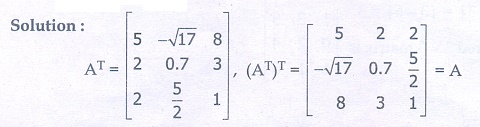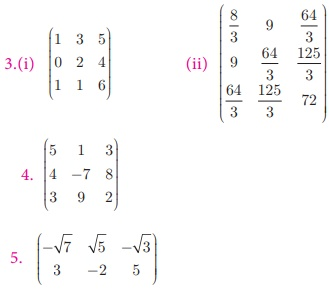Home | | Maths 10th Std | Exercise 3.16: Matrix

# Exercise 3.16: Matrix

Maths Book back answers and solution for Exercise questions - Mathematics : matrix: Exercise Problem Questions with Answer

Exercise 3.16

1. In the matrix A =, write

(i) The number of elements

(ii) The order of the matrix

(iii) Write the elements a22a23a24a34a43a44 .2. If a matrix has 18 elements, what are the possible orders it can have? What if it has 6 elements?3. Construct a 3 ×3 matrix whose elements are given by

(i) aij = |i − 2j| (ii) aij = (i+j)3/34. Ifthen find the transpose of A.5. Ifthen find the transpose of -A .6. If A =then verify (AT)T = A7. Find the values of x, y and z from the following equations1.(i) 16 (ii) 4 × 4 (iii) √7 , √3/2 , 5, 0, -11 , 1

2.1 × 18 , 2 × 9 , 3 × 6 , 6 × 3 , 9 × 2 , 18 × 1 and 1 × 6, 2 × 3, 3 × 2, 6 × 17.(i) 3,12,3 (ii) 4,2,0 or 2,4,0 (iii) 2,4,3

Tags : Problem Questions with Answer, Solution | Mathematics , 10th Mathematics : UNIT 3 : Algebra
Study Material, Lecturing Notes, Assignment, Reference, Wiki description explanation, brief detail
10th Mathematics : UNIT 3 : Algebra : Exercise 3.16: Matrix | Problem Questions with Answer, Solution | Mathematics